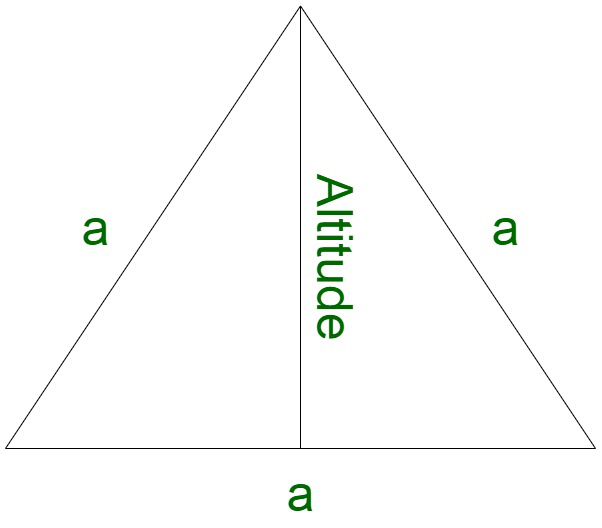# How to find the area of a triangle with one side given?

• Last Updated : 20 Nov, 2021

A triangle is a closed figure composed of three sides. An equilateral triangle is a geometrical 2D figure which has all three sides equal. All the edges subtend an angle of 60° at the corners. Therefore, the sum of all the angles of the triangle is 180°Properties of an equilateral triangle

• All three sides of an equilateral triangle are equal.
• All the three angles are equal i.e 60° each.
• A regular polygon has three equal sides.

### How to find the area of a triangle with one side given?

Solution:

Let us assume a to be the side of the triangle.

If we divide the bottom side in two parts, we get,

By Pythagoras theorem, the altitude can be obtained by,

Perpendicular2 + Base2 = Hypotenuse2

Perpendicular2 + (a/2)2 = a2

Perpendicular2 = a2 – (a/2)2

Perpendicular2 = 3a2/4

Taking square root,

Perpendicular = √3/2a

Now,

Area of triangle = 1/2 x Perpendicular x Base

= 1/2 x √3/2a x a

On solving, we obtain,

Area = a2(√3/4)

### Sample Questions

Question 1. Find the area of the equilateral triangular signboard of side 16 cm and also find the cost of painting the signboard at ₹2 per cm2?(Assume √3 = 1.732)

Solution:

Here we have to find the cost of painting an equilateral triangular signboard.

Given:

Side o

uilateral triangular signboard = 16 cm

First finding the area of the equilateral triangular sign board

As we know that

Area of equilateral triangle = √3/4 × a2

Here a = 16 cm

Area of equilateral triangle = √3/4 × 162

Area of equilateral triangle = √3/4 × 16 × 16

Area of equilateral triangle = 64√3

Now,

Finding the cost of painting

Given

Cost of painting = ₹2 per cm2

Cost of painting = Area of equilateral triangle × 2

Cost of painting = 64√3 × 2

Cost of painting = 128√3

Using √3 = 1.732 (Given)

Cost of painting = ₹221.696

Therefore,

Cost of painting the equilateral triangular signboard is ₹221.696.

Question 2. Find the area of an equilateral triangular park of side 32 m?<

ong>

Solution:

Here we have to find the area of the equilateral triangular park.

Here we are given that,

Side of the triangular park = 32 m

As we know that

Area of equilateral triangle = √3/4 × a2

Area of equilateral triangular park = √3/4 × a2

Here ‘a’ is the side of the equilateral triangular park is 32 m

Area of equilateral triangular park = √3/4 × 322

Area of equilateral triangular park = √3/4 × 32 × 32

Area of equilater

riangular park = √3 × 8 × 32

Area of equilateral triangular park = 256√3 m2

Therefore,

The area of the equilateral triangular park is 256√3 m2

Question 3. Find the cost of carpeting equilateral triangular yoga ground with the altitude 10 m and base 20 m at the rate of ₹20 pr m2?

Solution:

Here we have to find the cost of carpeting equilateral triangular yoga ground

Given:

Altitude = 10 m

Base = 20 m

As we know that

Area of equilateral triangle = 1/2 × base × height

Area of equilateral triangle = 1/2 × 20 × 10

Area of equilateral triangle = 100 m2

Now finding the cost of carpeting

Cost of carpeting yoga ground at ₹20 pr m2

Cost of carpeting yoga ground = Area of yoga ground × ₹20

Cost of carpeting yoga ground = 100 × ₹20

Cost of carpeting yoga ground = ₹2000

Therefore,

The cos

carpeting the yoga ground is ₹2000.

Question 4. Find the area of an equilateral triangle having a perimeter of 18 m?

Solution:

Here we have to find the area of the equilateral triangle

Given:

Perimeter of equilateral triangle = 18 m

The Perimeter of equilateral triangle = Side + Side + Side

As all the sides of the equilateral triangle are equal

Perimeter of equilateral triangle = 3 × Sid

>

18 = 3 × Side

Side = 18/3

Side = 6 m

Side of the equilateral triangle is 6 m

Further,

Finding the area of the equilateral triangle

Area of equilateral triangle = √3/4 × a2

a =  side of the equilateral triangle

Area of equilateral triangle = √3/4 × 62

Area of equilateral triangle = √3/4 × 6 × 6

Area of equilateral triangle = 9√3 m2

Question 5. If the side of an equilateral triangle is 10 m and its area is 75 m2  then find the he

of the equilateral triangle?

Solution:

Here we have to find the height of the equilateral triangle

Given:

Side of equilateral triangle = Base of equilateral triangle = 10 m

Area of equilateral triangle = 75 m2

As we know that

Area of equilateral triangle = 1/2 × base × height

75 = 1/2 × 10 × height

75 = 5 × height

Height = 75/5

Height = 15 m

Therefore,

The height of equilateral triangle is 15 m.

My Personal Notes arrow_drop_up